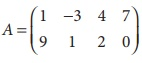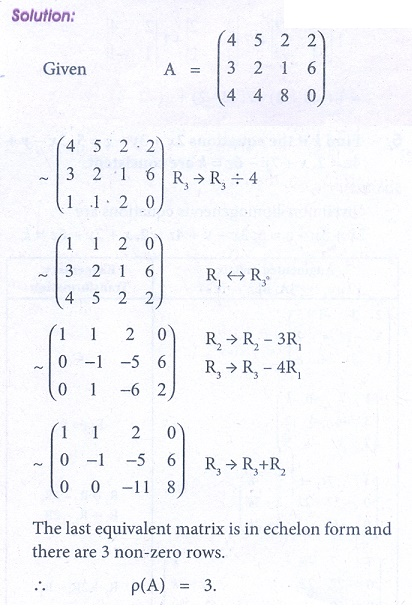Home | | Business Maths 12th Std | Miscellaneous problems

# Miscellaneous problems

Business Maths and Statistics : Applications of Matrices and Determinants: Miscellaneous problems with Answers, Solution and Explanation

Miscellaneous problems

1. Find the rank of the matrix2. Find the rank of the matrix A =3. Find the rank of the matrix A =4. Examine the consistency of the system of equations: x y + z = 7 , x + 2 y + 3z = 18, y + 2z = 6 .5. Find k if the equations 2x + 3y ã z = 5 , 3x ã y + 4z = 2 , x + 7 y ã 6z = k are consistent.6. Find k if the equations x + y + z = 1, 3x ã y ã z = 4, x + 5y + 5z = k are inconsistent.

1.7. Solve the equations x + 2 y + z = 7, 2x ã y + 2z = 4, x + y ã 2z = ã1 by using Cramerãs rule8. The cost of 2kg. of wheat and 1kg. of sugar is ã¿100. The cost of 1kg. of wheat and 1kg. of rice is ã¿80. The cost of 3kg. of wheat, 2kg. of sugar and 1kg of rice is ã¿220. Find the cost of each per kg., using Cramerãs rule.9. A salesman has the following record of sales during three months for three items A,B and C, which have different rates of commission.Find out the rate of commission on the items A,B and C by using Cramerãs rule.10. The subscription department of a magazine sends out a letter to a large mailing list inviting subscriptions for the magazine. Some of the people receiving this letter already subscribe to the magazine while others do not. From this mailing list, 60% of those who already subscribe will subscribe again while 25% of those who do not now subscribe will subscribe. On the last letter it was found that 40% of those receiving it ordered a subscription. What percent of those receiving the current letter can be expected to order a subscription?1. ü(A)= 2

2. ü(A) = 3

3. ü(A) = 3

4. The given system is inconsistent and has no solution.

5. k = 8.

6. The equations are inconsistent when k assumes any real value other than 0.

7. x=1,  y = 2 , and z = 2

8. Cost of wheat is ã¿30/kg, Cost of sugar is ã¿40/kg and Cost of rice is ã¿50/kg.

9. The rates of commission for A,B and C are ã¿2, ã¿4 and ã¿11 respectively

10. 39%

Tags : Applications of Matrices and Determinants , 12th Business Maths and Statistics : Chapter 1 : Applications of Matrices and Determinants
Study Material, Lecturing Notes, Assignment, Reference, Wiki description explanation, brief detail
12th Business Maths and Statistics : Chapter 1 : Applications of Matrices and Determinants : Miscellaneous problems | Applications of Matrices and Determinants